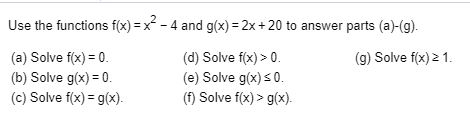# Use the functions f(x)-x-4 and g(x) 2x +20 to answer parts (a)-(g).(a) Solve fx) 0(b) Solve g(x) 0(c) Solve f(x) g(x)(d) Solve f(x)> 0(e) Solve g(x)s0(f) Solve f(x)> g(x)(g) Solve fx)2 1

Question

Use the function f(x)=x^2-4 and g(x)=2x+20 answer parts (a)-(g).

(a) solve f(x)=0
(b) solve g(x)=0
(c) solve f(x)=g(x)
(d) solve f(x)>0
(e) solve g(x)<_0
(f) solve f(x)>g(x).
(g) solve f(x)>-1help_outlineImage TranscriptioncloseUse the functions f(x)-x-4 and g(x) 2x +20 to answer parts (a)-(g). (a) Solve fx) 0 (b) Solve g(x) 0 (c) Solve f(x) g(x) (d) Solve f(x)> 0 (e) Solve g(x)s0 (f) Solve f(x)> g(x) (g) Solve fx)2 1 fullscreen
check_circle

Step 1

Since, There are multiple number of subparts in the question, we are answering only the first three subparts.

(a) The given functions are

Step 2

Substitute f(x)=0 and solve for x as shown below:

Step 3

(b) Substitute g(x)=0 and solve for x ...

### Want to see the full answer?

See Solution

#### Want to see this answer and more?

Solutions are written by subject experts who are available 24/7. Questions are typically answered within 1 hour.*

See Solution
*Response times may vary by subject and question.
Tagged in

### Algebra